Examples

Chapter 10 Class 11 Straight Lines
Serial order wise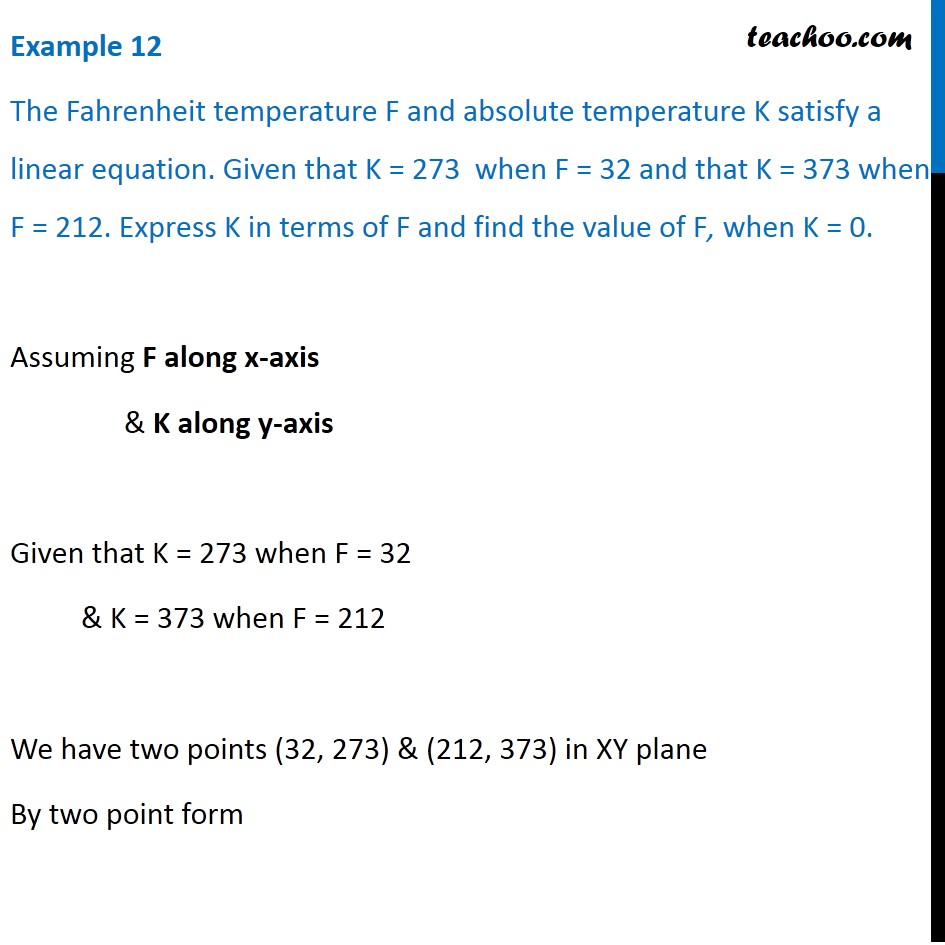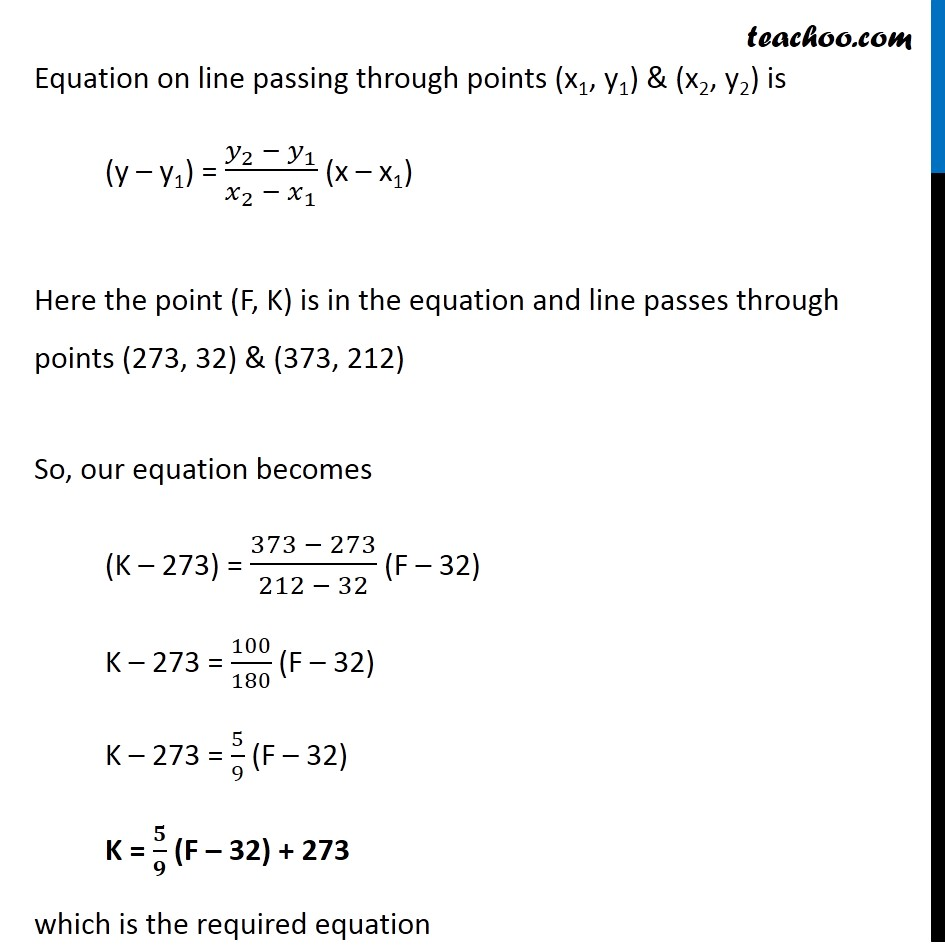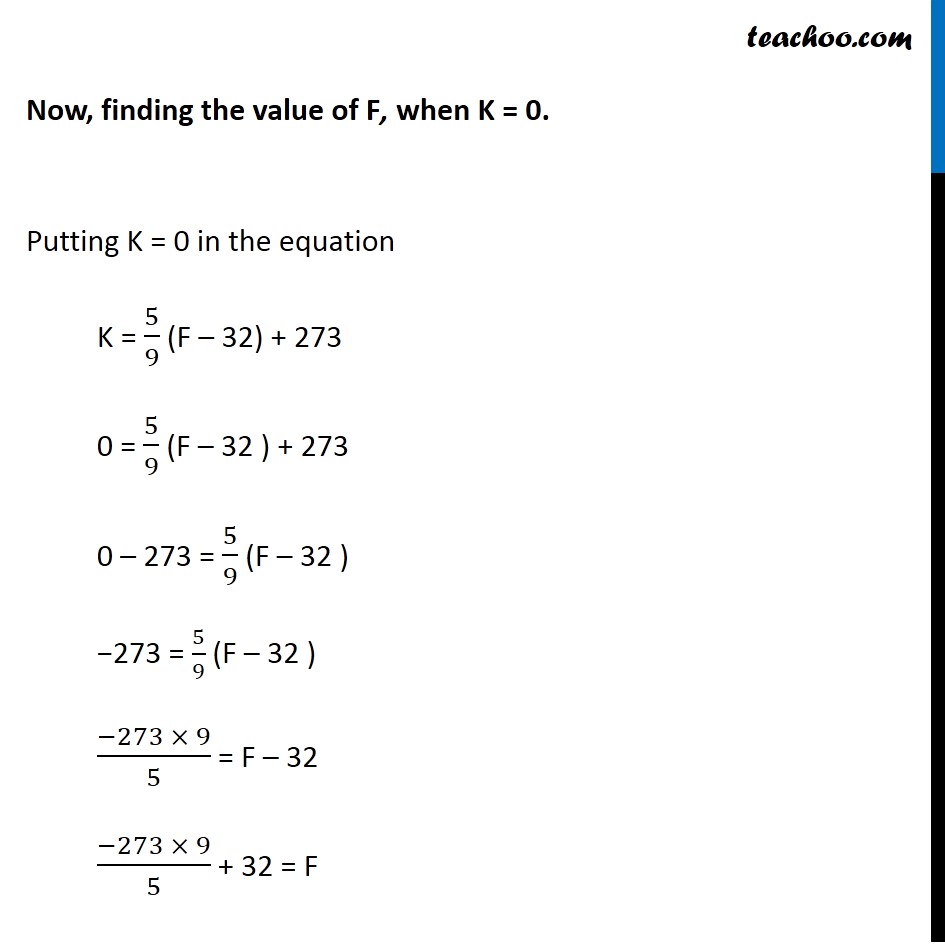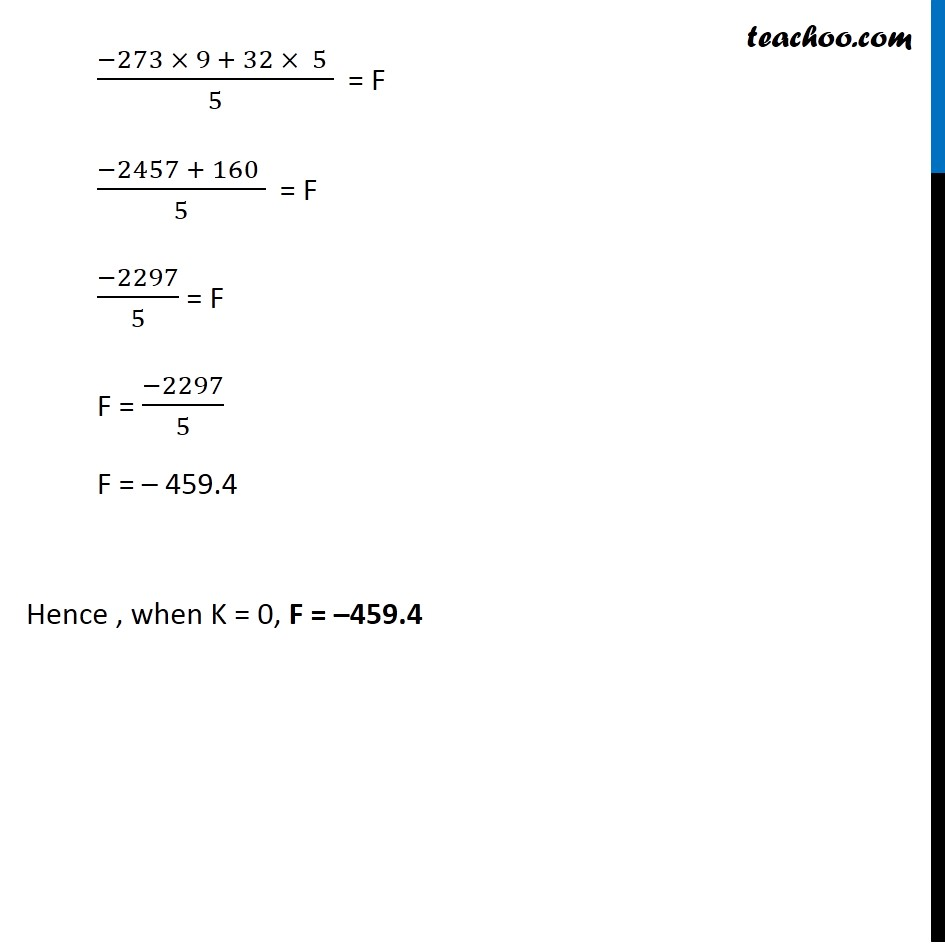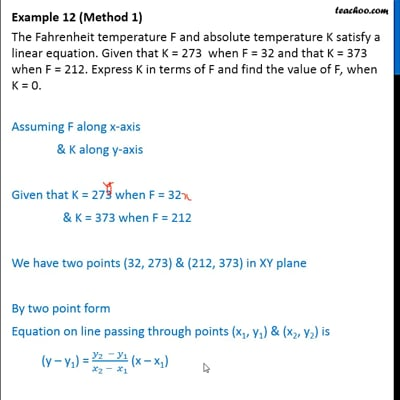This video is only available for Teachoo black users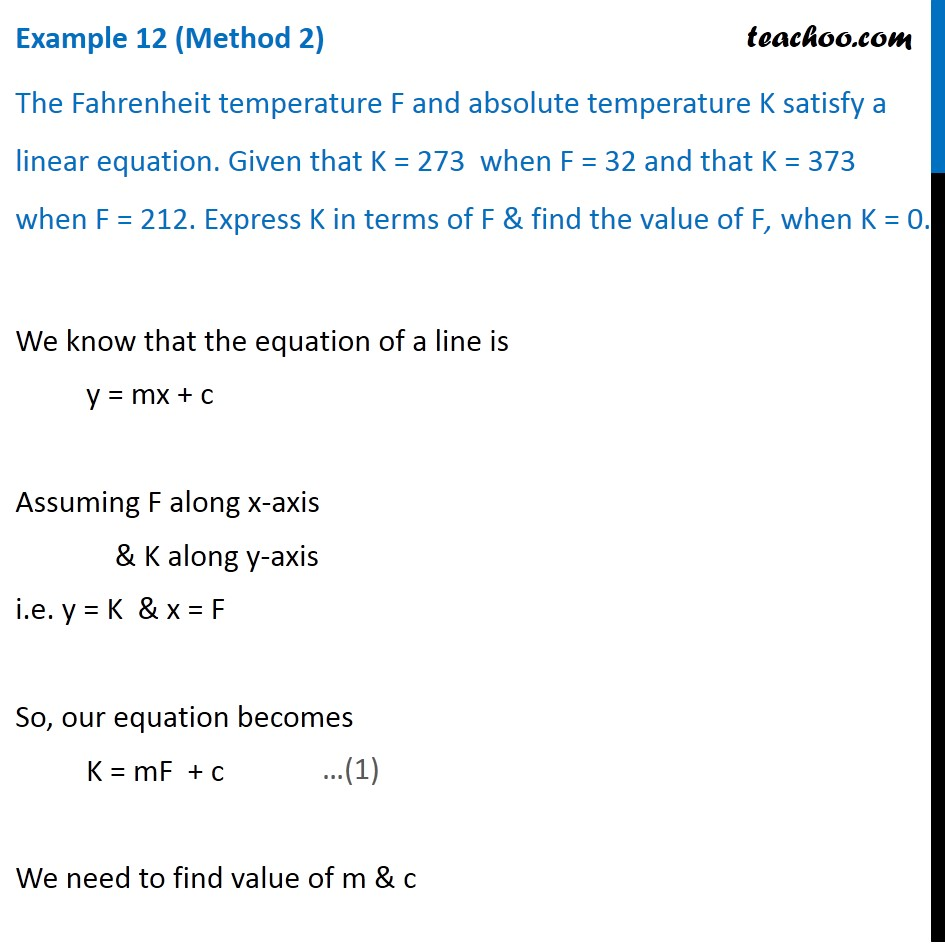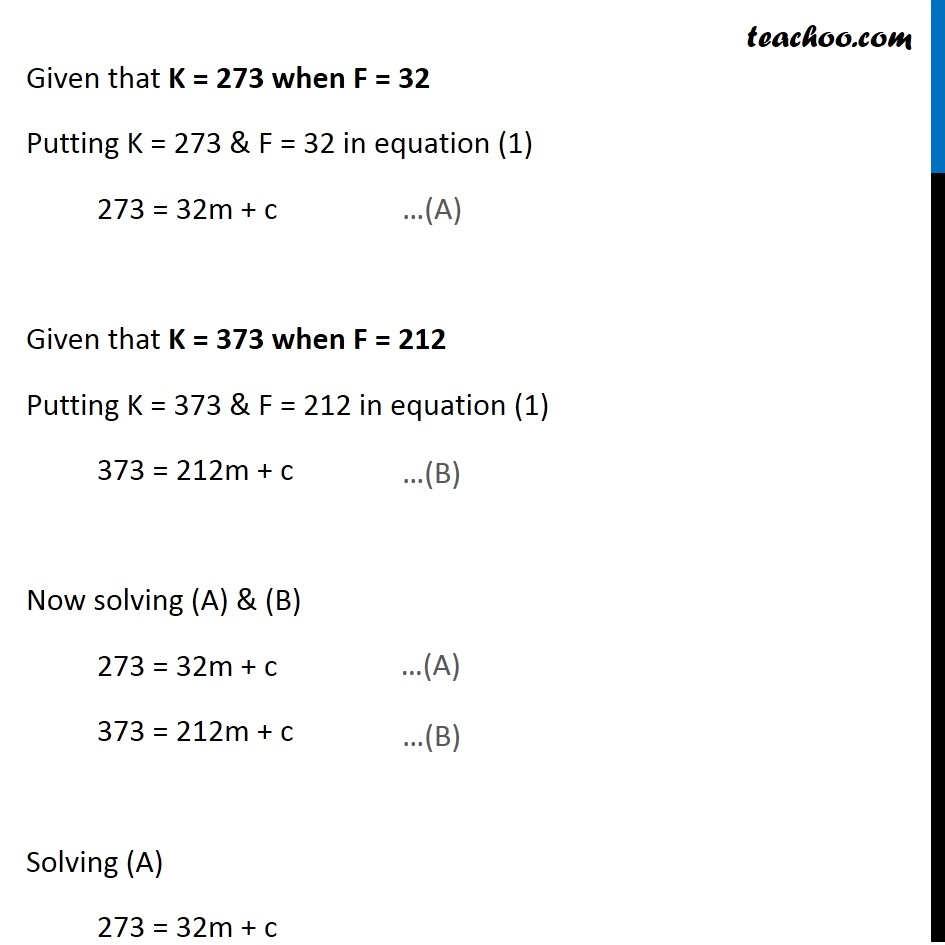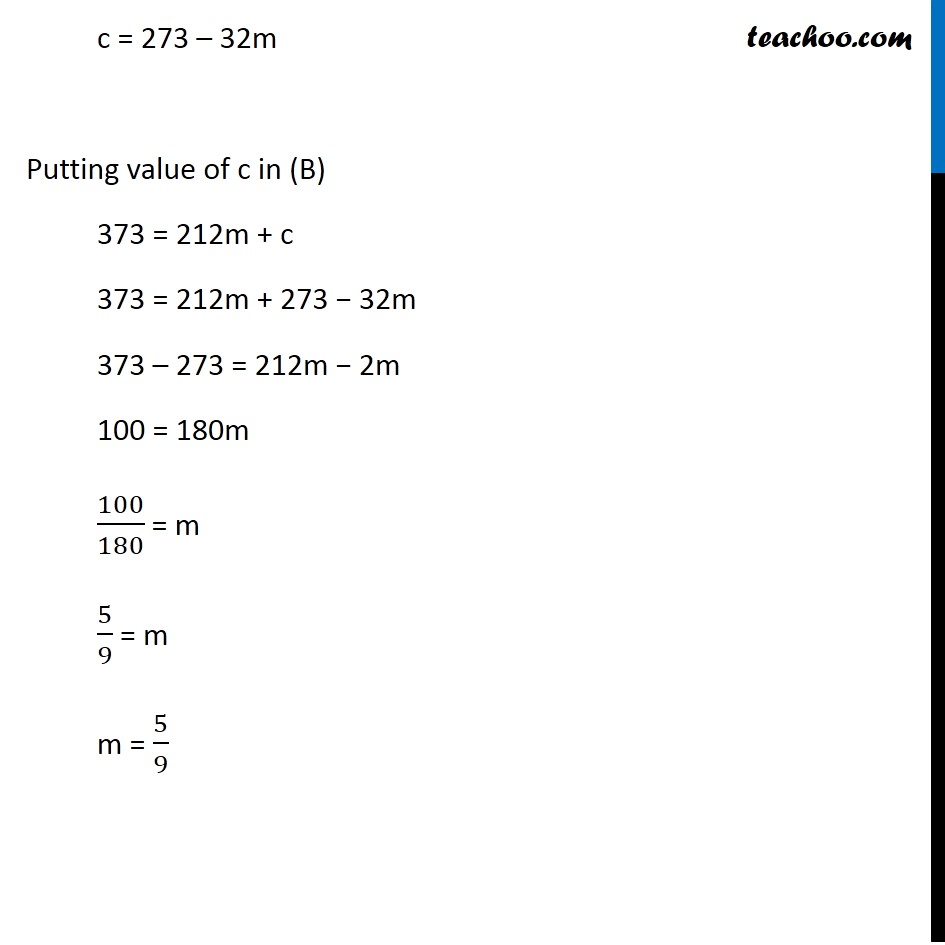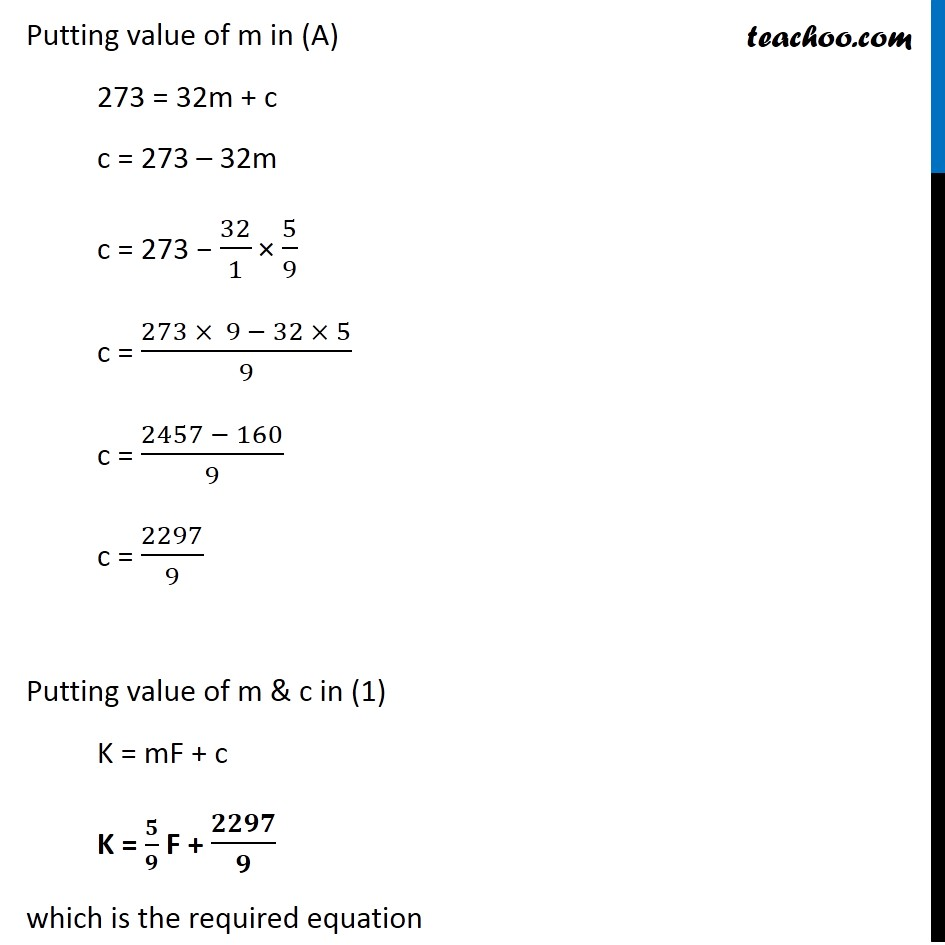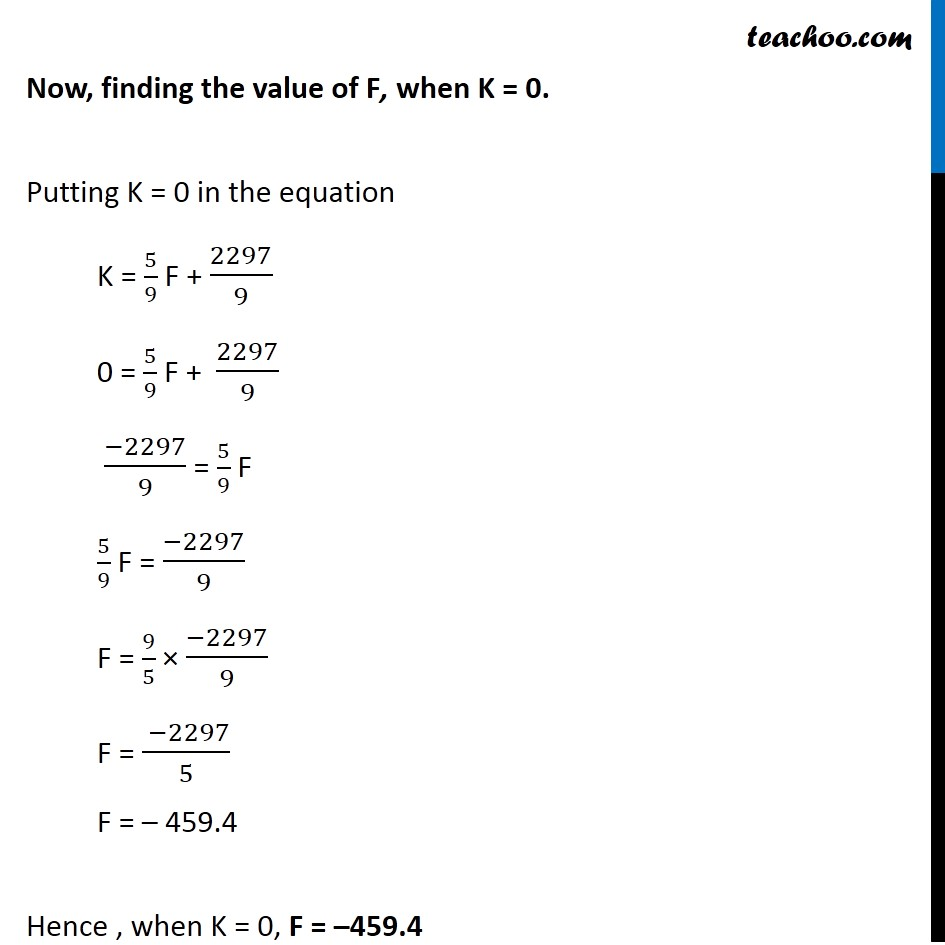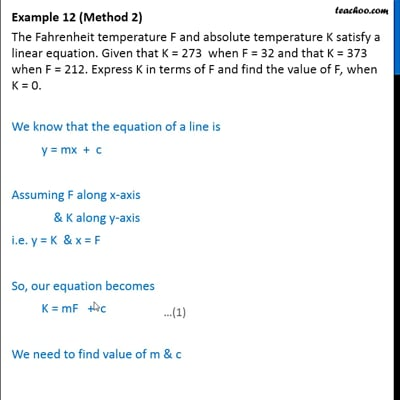This video is only available for Teachoo black users

Solve all your doubts with Teachoo Black (new monthly pack available now!)

### Transcript

Example 12 The Fahrenheit temperature F and absolute temperature K satisfy a linear equation. Given that K = 273 when F = 32 and that K = 373 when F = 212. Express K in terms of F and find the value of F, when K = 0. Assuming F along x-axis & K along y-axis Given that K = 273 when F = 32 & K = 373 when F = 212 We have two points (32, 273) & (212, 373) in XY plane By two point form Equation on line passing through points (x1, y1) & (x2, y2) is (y – y1) = (𝑦_2 − 𝑦_1)/(𝑥_2 − 𝑥_1 ) (x – x1) Here the point (F, K) is in the equation and line passes through points (273, 32) & (373, 212) So, our equation becomes (K – 273) = (373 − 273)/(212 − 32) (F – 32) K – 273 = 100/180 (F – 32) K – 273 = 5/9 (F – 32) K = 𝟓/𝟗 (F – 32) + 273 which is the required equation Now, finding the value of F, when K = 0. Putting K = 0 in the equation K = 5/9 (F – 32) + 273 0 = 5/9 (F – 32 ) + 273 0 – 273 = 5/9 (F – 32 ) −273 = 5/9 (F – 32 ) (−273 × 9)/5 = F – 32 (−273 × 9)/5 + 32 = F (−273 × 9 + 32 × 5 )/5 = F (−2457 + 160 )/5 = F (−2297)/5 = F F = (−2297)/5 F = – 459.4 Hence , when K = 0, F = –459.4 Example 12 (Method 2) The Fahrenheit temperature F and absolute temperature K satisfy a linear equation. Given that K = 273 when F = 32 and that K = 373 when F = 212. Express K in terms of F & find the value of F, when K = 0. We know that the equation of a line is y = mx + c Assuming F along x-axis & K along y-axis i.e. y = K & x = F So, our equation becomes K = mF + c We need to find value of m & c Given that K = 273 when F = 32 Putting K = 273 & F = 32 in equation (1) 273 = 32m + c Given that K = 373 when F = 212 Putting K = 373 & F = 212 in equation (1) 373 = 212m + c Now solving (A) & (B) 273 = 32m + c 373 = 212m + c Solving (A) 273 = 32m + c c = 273 – 32m Putting value of c in (B) 373 = 212m + c 373 = 212m + 273 − 32m 373 – 273 = 212m − 2m 100 = 180m 100/180 = m 5/9 = m m = 5/9 Putting value of m in (A) 273 = 32m + c c = 273 – 32m c = 273 − 32/1 × 5/9 c = (273 × 9 − 32 × 5)/9 c = (2457 − 160)/9 c = 2297/9 Putting value of m & c in (1) K = mF + c K = 𝟓/𝟗 F + 𝟐𝟐𝟗𝟕/𝟗 which is the required equation Now, finding the value of F, when K = 0. Putting K = 0 in the equation K = 5/9 F + 2297/9 0 = 5/9 F + 2297/9 (−2297)/9 = 5/9 F 5/9 F = (−2297)/9 F = 9/5 × (−2297)/9 F = ( −2297)/5 F = – 459.4 Hence , when K = 0, F = –459.4